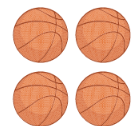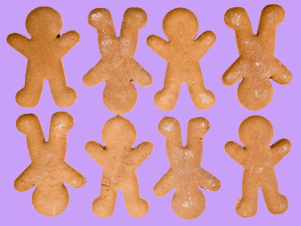Number Forms
Place Value
Even or Odd
Let's Compare

100

Write 372 in expanded form.

300 + 70 + 2

100

In the number four hundred seventy-three, which digit is in the tens place?

473

7

100

Is this an even amount or an odd amount?odd

100

Is this equation true or false

752 < 751

False

100

30

20

+  10

60

200

Write 200 + 50 + 1 in word form.

two hundred fifty-one

200

What is the value of the underlined digit?

792

90

200

Jack has 8 marbles. Does he have an or or even number of marbles

an even number of marbles

200

What symbol makes this number sentence true?

650                665

A) >

B) <

C) =

B. <

42

7

+10

59

300

Write 507 in expanded form.

500 + 7

300

What digit is in the hundreds place?

637

6

300

Marcus has 7 strawberries. He says its an even numbers of strawberries. Is he correct?

No, seven is odd.

300

What symbol makes the number sentence true?

487 _____ 487

a. <

b. >

c. =

c. =

23

12

+ 31

66

400

Write the standard form  300 + 70 + 4

374

400

What is the value of the digit in the hundreds place?

777

700

400

Is this an Even or Odd number of cookies?Even

400

What symbol makes the number sentence true?

921 _____ 924

a. <

b. >

c. =

a. <

45

31

+ 19

85

500

Write 4 + 800 + 30 in standard form

834

500

Use the digits 3, 2,  and 6 to create a number with the greatest value.

632

500

Which statement about the number 21 is true

a. It is even because 21  can be made into pairs with none left over.

b. It is odd because when you make pairs you have one left over.

b. It is odd because when you make pairs you have one left over.

500

Which number sentence is true?

A) 428   >   824

B) 547   <   543

C) 352   <   352

D) 863   >   836

D) 863   >   836

78

96

+ 89

263